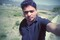Join the social network of Tech Nerds, increase skill rank, get work, manage projects...

• # Smart ways of testing@lalit.bisht• 0
• 0
• 0
• 0
• 0
• 0
• 0
• 0
• 139
Comment on it

below are few techniques

Equivalence partitioning: Dividing the data into a range of values and selecting one input data value from each range is called Equivalence Partitioning. This is a black box test designing technique used to calculate the quality of test cases and which can be applied to all levels of testing from unit, integration, system testing and so forth.

testing all the values is not possible, because if try to do this the number of test cases would extend in large numbers. In this method, input data is divided into different classes, each class is representing the input criteria from the equivalence class. We then select one input from each class.

we can use this technique to reduce an infinite number of test cases to a finite number, while ensuring that the selected test cases are still effective test cases which will cover all possible scenarios..

Lets take a very basic and simple example to understand the Equivalence partitioning concept:

If one application is accepting input range from 1 to 100, using equivalence class we can divide inputs into the classes, for example, one for valid input and another for invalid input and design one test case from each class.

In this example test cases are chosen as below:

One is for valid input class i.e. selects any value from input between ranges 1 to 100. So here we are not writing hundreds of test cases for each value. Any one value from this equivalence class should give you the same result. One is for invalid data below lower limit i.e. any one value below 1. One is for invalid data above upper limit i.e. any value above 100.

Boundary value analysis: For the most of the cases we observed the errors coming in extreme ends of the input values, so these extreme values might be the start of value and end of values are called Boundary values and to analyses of the values is Boundary value analysis, we also say this as "range checking"

this technique is a black box test design technique and it is used to find the errors at boundaries of input domain rather than finding those errors in the center of input.

Equivalence Partitioning and Boundary value analysis both are linked to each other and can be used together at all levels of testing. Based on the edges of the equivalence classes, test cases can then be derived.

Each boundary has a valid boundary value and an invalid boundary value. Test cases are designed based on the both valid and invalid boundary values. Typically, we choose one test case from each boundary.

Finding defects using Boundary value analysis test design technique is very effective and it can be used at all test levels. You can select multiple test cases from valid and invalid input domains based on your needs or previous experience but remember you do have to select at least one test case from each input domain.

Lets take same above example to understand the Boundary value analysis concept:

One test case for exact boundary values of input domains each means 1 and 100. One test case for just below boundary value of input domains each means 0 and 99. One test case for just above boundary values of input domains each means 2 and 101.

## 0 Comment(s)

OR
OR
Register

OR
Fill out the form below and instructions to reset your password will be emailed to you:

• Hire
• Post Projects

### Post Projects

• All at 0 Cost ....
• Post Tech Job
• Select Best Bidder
• Track the Project
• Approve Work and Pay safely
• Browse Nerds
• Work
• Find Projects Find Projects
• UI Design and UX
• Software Engineering
View more...
View less...
• Marketing
• General
• Manage
• Company Company

### Manage Company

• All at 0 Cost ....
• Manage Company and Employee Profiles
• Company wide Employee Productivity Reports
• Knowledge Sharing and Collaboration Tools
• Get Sales Lead and Bid for Tech Projects
• Send Invoices and Receive Payment Safely
• Learn
• Nerd Digest Nerd Digest
• UI Design and UX
• Software Engineering
View more...
View less...
• Marketing
• General
• Tech Q & A Tech Q & A
• UI Design and UX
• Software Engineering
View more...
View less...
• Marketing
• General